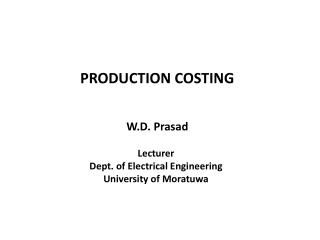Download PresentationPRODUCTION COSTING

# PRODUCTION COSTING - PowerPoint PPT PresentationDownload Presentation## PRODUCTION COSTING

- - - - - - - - - - - - - - - - - - - - - - - - - - - E N D - - - - - - - - - - - - - - - - - - - - - - - - - - -
##### Presentation Transcript

1. PRODUCTION COSTING W.D. Prasad Lecturer Dept. of Electrical Engineering University of Moratuwa

2. Introduction • Electricity generation system planning requires minimization of the total cost of supplying the demand during a specified period of time. • Short term, Medium term or Long term. • Medium term and Long term planning • initial investment cost + production costs • Short term planning • production cost only

3. Load and Generator Models • Production costing includes probabilistic treatment of the system load and the generation unit availability in almost all planning models. • Chronological load curve Load duration curve • Chronological load curve is modified with a plot of load versus the duration that the system load exceeds that load level. • This curve can be converted to a probability curve, F(x) by dividing the horizontal axis (x- axis) by the total duration of the chronological load profile, T and rearranging the axes (Load Duration Curve, LDC).

4. pi - Availability qi -FOR 0 Ci ; Ci = Capacity Load and Generator Models Cont… • Generators are normally represented by a two-state model where either a generating unit is available at its full capacity or not available. • Probability associated with the state where the unit is not available is called Forced Outage Rate (FOR).

5. Production Cost Calculation • Generators are first ranked according to their average incremental costs so that the units with the lower costs are placed at the top of the list (Merit Order). • These units are now gradually loaded onto the LDC in merit order. • After loading each generator the Effective Load Duration Curve (ELDC), F ican be obtained. • The area under each of these ELDCs multiplied by the normalizing value, T, directly indicates the energy not served in the system. • Unserved energy (UEi) after loading the generator i is given by, Where T is the total duration

6. Production Cost Calculation Cont… • Once the unserved energies are known the difference in unserved energies before and after loading a generator can be used to obtain the energy served by that generator. • Energy produced by generator i, Eiis given by, • Corresponding production cost, Costi is given by where ICi is the incremental cost of generator i • Total production cost, TC is given by Where ng is the number of generator units

7. Multiple Availability States of Generators • In most practical circumstances some of the generation units are likely to be deliberately operated at output levels below their full capacities during operation. • Consider a generating unit model with two availability states. • New ELDC will be • In the case of a generator with multi-level availability states where n is the no. of availability levels

8. System Unserved Energy and Loss of Load Probability • After loading all the generating units onto the load curve there will be a final ELDC left behind. • Loss of Load Probability (LOLP) is the probability that the system generation is not able to supply the system load either fully or partially. This can be directly obtained from the final ELDC.

9. The total energy left to be served after loading all the generating units is called the Expected System Unserved Energy. • Average cost of losses due to the power supply failures is called the Value of Lost Load (VLL) which is given in Rs/ kWh not supplied. • System planners need to add new generating units into the system until the following condition is satisfied.

10. Example • [a] Determine LOLP, EUE and total production cost if the system load given in Table 1 is supplied with generators in Table 2. Table 1: Load Variation Table 2: Generator Data